edHelper subscribers - Create a new printable

Number of Pages
 Select the number of pages: 1 page 2 pages 3 pages 4 pages

Include an answer key (answer keys will be at the end of the printable)

Sample edHelper.com - Math Review Worksheet

 Name _____________________________Date ___________________
Algebra
Solve each equation by factoring.
1.
 17x - 79 = -4x2 - 2

2.
 -75x2 + 290x = 240

3.
 13
x2 - 3x +
14
 23
=  2x

Complete and write in lowest terms.
4.
 2k5
-
 9k15

5.
 4c7
-
 8c28

6.
 7b6
-
 5b - 1224

Graph each equation.
7.
 y = 4 x - 8

 8 2y = -6

Solve each system by graphing. Find the vertices of the graph.
 9 3x - 2y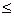55x + 2y33x - 4y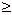-19x - 8y-13
 10 3x - y44x - y172x - y = 57x - 5y20
 11 2x + 3y5x + y4x - 3y-203y9

Complete.
 12 Find the sum of 3j2 + 12j - 4 and 6j2 + 11j - 7.
13.
 The equation a + k = k + a
is an example of which of the following?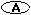the communitive law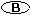distributive property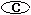identity property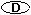the associative law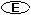inverse property

State the inverse of each relation. Is the inverse a function?
 14 {(-11, -10), (-12, -9), (9, -12), (16, 6), (18, -2), (-5, 3)}
 15 {(4, 7), (13, -9), (-10, -9), (-19, -9)}
 16 {(-5, -4), (18, -5), (-15, -2), (-9, -9), (11, 0)}

Simplify.
 17 (-2a6)(6a2)
 18 (-2k6)(-4k5n6)(-11k5)
 19 (-10s6z3)(-8s6)

Factor out the GCF from each polynomial.
 20 -26x4 + 234x2
 21 -18x4 + 16x3
 22 5x6 + 12x4

Sample
This is only a sample worksheet.

edHelper subscribers - Create a new printable

Number of Pages
 Select the number of pages: 1 page 2 pages 3 pages 4 pages